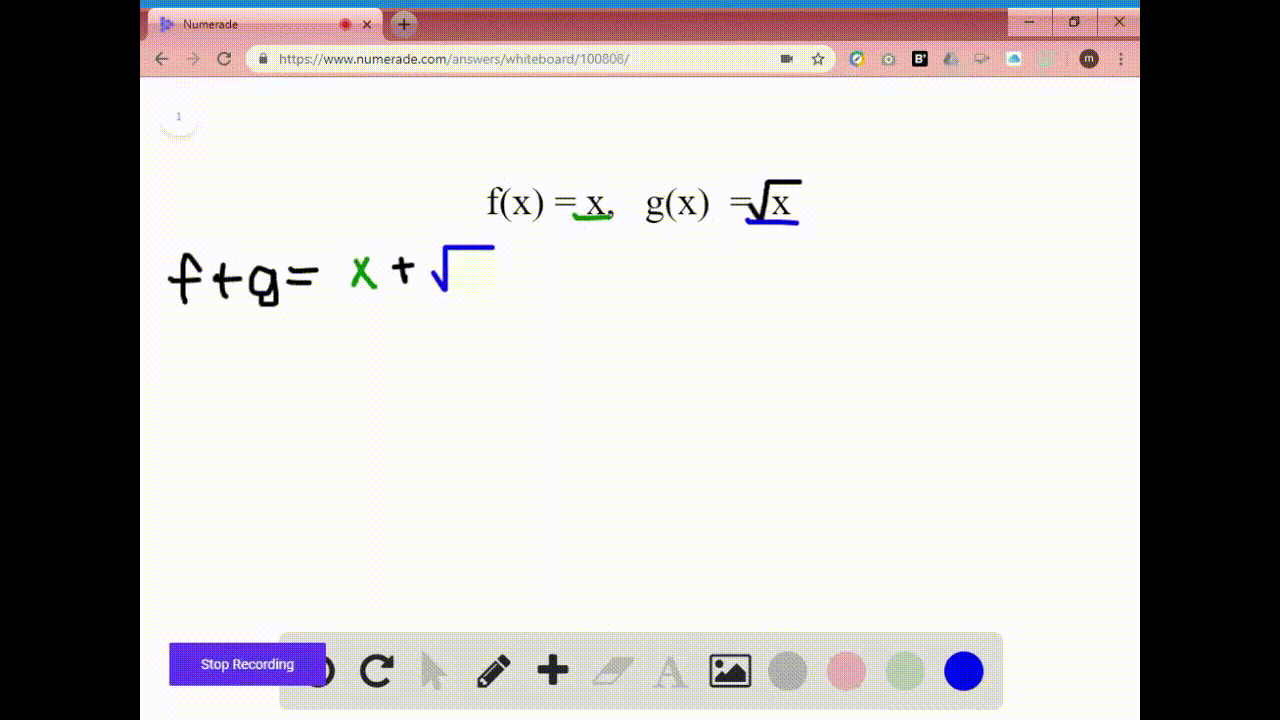Enroll in one of our FREE online STEM summer camps. Space is limited so join now!View Summer Courses### $7-16=$ Combining Functions $\quad$ Find $f+g, f-… 02:57Syracuse University Need more help? Fill out this quick form to get professional live tutoring. Get live tutoring Problem 7$7-16=$Combining Functions$\quad$Find$f+g, f-g, f g,$and$f / g\$ and their domains.}
$$f(x)=x, \quad g(x)=2 x$$

## Discussion

You must be signed in to discuss.

## Video Transcript

So in this problem, forgiven that f of X is equal to X and G of X is equal to two X. So what we want to find is each of the following combinations of functions as well as their domains. Well, let's start with F plus G. Well, that means to add my F function, which is X to my GI function, which is to ex well X plus two X is equal to three X. So now for the domain. Well, I can pull again any value I want in for X and get a value for why. Therefore, my domain goes from negative infinity, the positive infinity Now for F minus. Gee, well, that's means to take my F function, which is X and subtract my GI function, which is to ex well X minus two x is negative X and now for the domain. Well again, I can pull get any value for exit I want and I will get any value for why so Therefore, our domain again is negative for beneath deposit infinity. All right, Next we have f times G. That means to take my F function, which is X and multiply it by a bi G function which is to acts well. X times two x is two x squared Now for the domain again, I can plug in any value that I want for X and I will not have any value. And I will be able to excuse me, get any value for why therefore our domain will be again Negative Infinity, the positive infinity Now for F divided by G, that means to take my F function, which is X and divide it by my GI function, which is to acts well X divided by two ex is just 1/2 now for the domain. Here's where we got to be careful, because if you just look at what it reduces to, you'd have 1/2 and you would say, Oh, well, I don't have any issues. However, our function really starts right here, but we have a fraction and our fraction can't have zero in the denominator. So when with our denominator equal zero, well, that would happen when two X is equal to zero, in other words, happens when X is equal to zero, so zero cannot be in our domain. However, every other value can be, so our domain will go from negative infinity to zero and then from zero to infinity